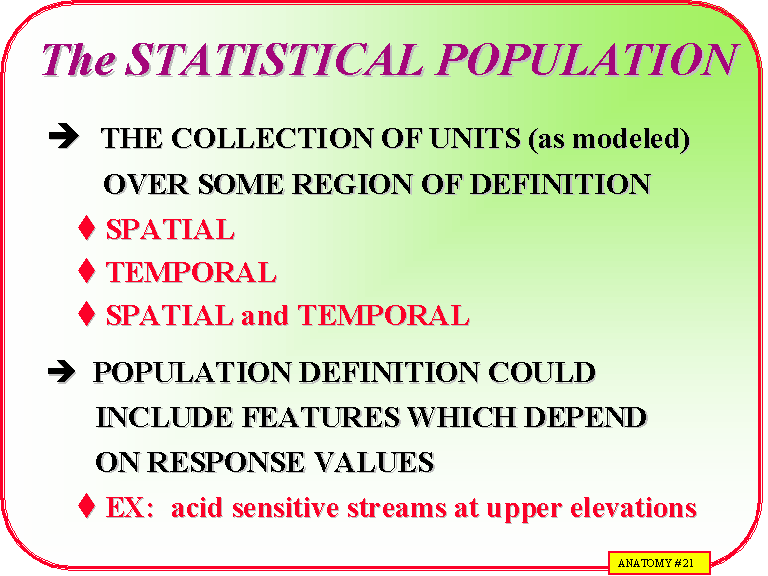# Concept of Statistical Population

In statistics, a population is a set of similar items or events which is of interest for some question or experiment. A statistical population can be a group of existing objects (e.g. the set of all stars within the Milky Way galaxy) or a hypothetical and potentially infinite group of objects conceived as a generalization from experience (e.g. the set of all possible hands in a game of poker). A common aim of statistical analysis is to produce information about some chosen population.

In statistical inference, a subset of the population (a statistical sample) is chosen to represent the population in a statistical analysis. The ratio of the size of this statistical sample to the size of the population is called a sampling fraction. It is then possible to estimate the population parameters using the appropriate sample statistics.

In statistics, the term population is used to describe the subjects of a particular study—everything or everyone who is the subject of a statistical observation. Populations can be large or small in size and defined by any number of characteristics, though these groups are typically defined specifically rather than vaguely—for instance, a population of women over 18 who buy coffee at Starbucks rather than a population of women over 18.

Statistical populations are used to observe behaviors, trends, and patterns in the way individuals in a defined group interact with the world around them, allowing statisticians to draw conclusions about the characteristics of the subjects of study, although these subjects are most often humans, animals, and plants, and even objects like stars.

 Overview: Statistical Population Function Statistical Analysis Definition A set of observations that share a property or set of properties. Example Coffee drinkers in France Value Targeting a set of data for the purposes of analysis. Related Techniques Statistical Model Probability Distribution## 2 thoughts on “Concept of Statistical Population”

error: Content is protected !!